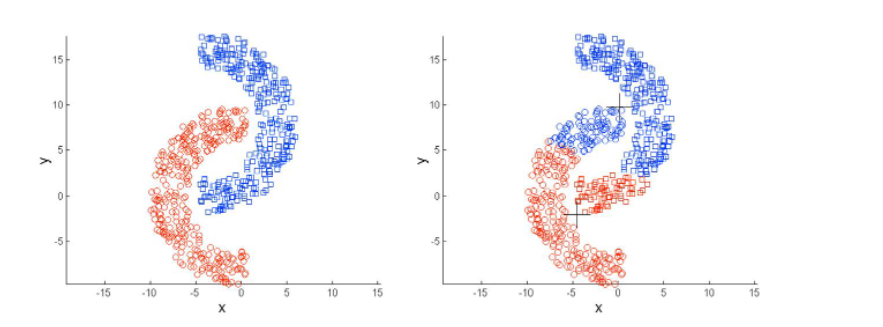# K Means Clustering

dougieduk·2022년 4월 3일
0

# Concept

• an unsupervised learning algorithm that will attempt to group similar clusters together in the data.

• Example problems
- Cluster Customers based on Features
- Market Segmentation
- Identify similar physical groups

• Goal : divide data into distinct groups such that observations within each group are similar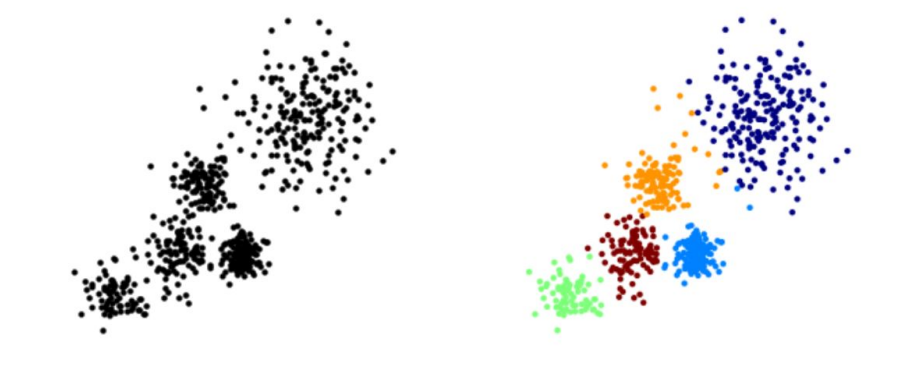# Process

It's an EM (Expectation Maximization) Method

• Choose a number of Clusters “K”
• Randomly assign each point to a cluster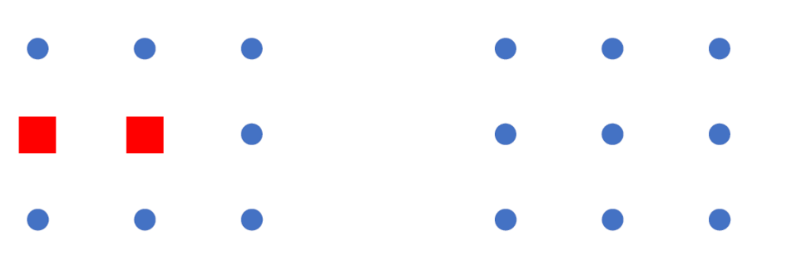• Until clusters stop changing, repeat the following:
- Assign each data point to the cluster for which the centroid is the closest (Expectation)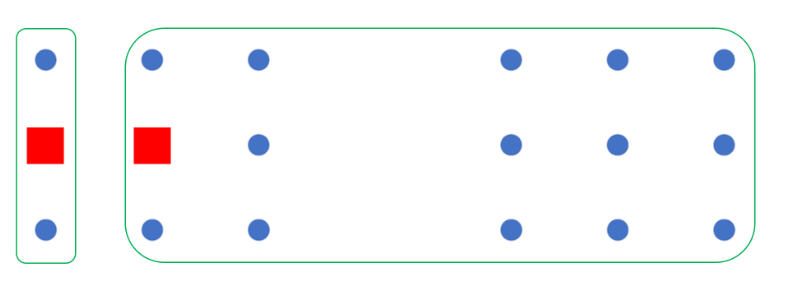• For each cluster, compute the cluster centroid by taking the mean vector of points in the cluster (Maximization)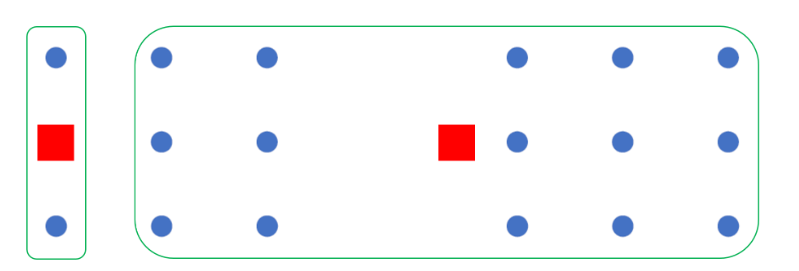- If the results do not change or the model reaches the maximum number of iterations, finish training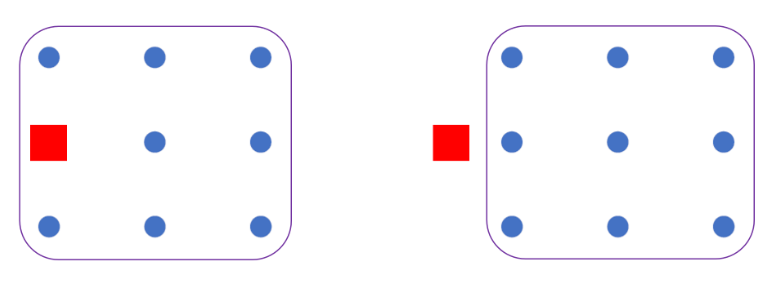# Choosing K

• There is no set way to choose K
• One way is elbow method
- compute the sum of squared error (SSE) for some values of k (for example 2, 4, 6, 8, etc.).
- SSE : the sum of the squared distance
between each member of the cluster and its centroid.
• k ↑ → SSE↓
- this is because when the number
of clusters increases, group sizes and distortion is also smaller
• Choose k value where SSE decreases abruptly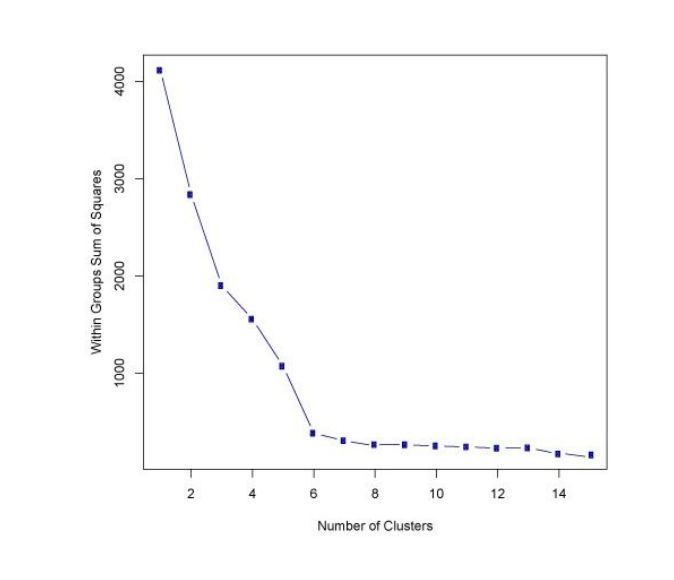# Pros & Cons

• Cons
- initial clustering might distort the result
- if the size of the clusters differ greatly, the algorithm might not work well
- if the desnsity of the clusters differ greatly, the algorithm might not work well
- if the distribution is unique, the algorithm might not work well